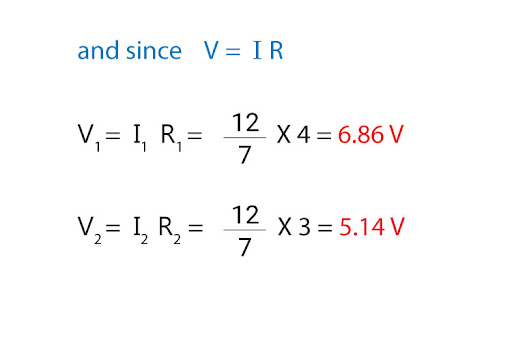# "Find current and voltage across resistors R1 and R2, when they connected in parallel and in series. A 12V battery is connected, R1=4Ω and R2=3Ω."

Let us first arrange our resistors R1 and R2 in parallel, and see what we get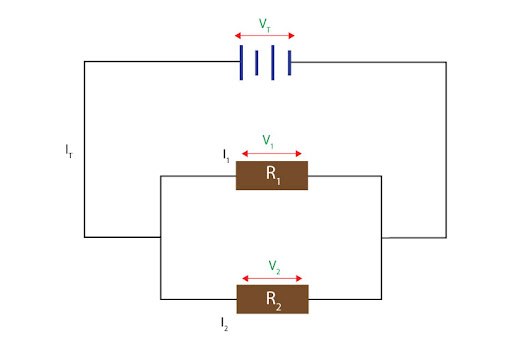Here, we have to acknowledge that since R1 and R2 are sharing the same two junctions that the battery is sharing, therefore R1 and R2 receive the same potential difference as the battery’s emf Vt: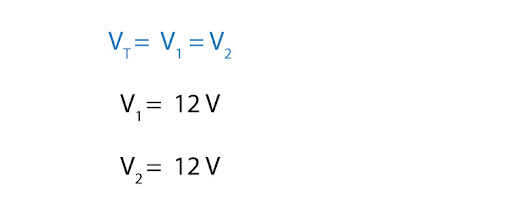Now we can easily calculate the individual current values flowing through R1 and R2: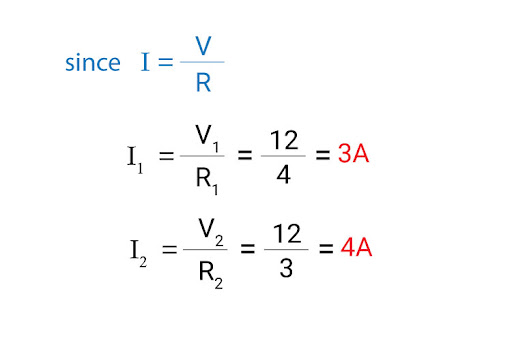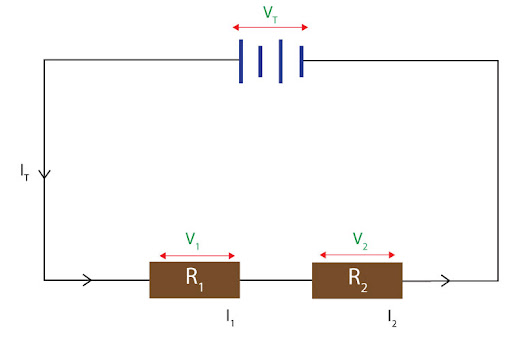Here, we see that both R1 and R2 receive the same value of current as the total current; on the other hand, potential differences will divide. So we will start by finding total resistance (i.e. an arithmetic addition of R1 and R2), and find current as follows: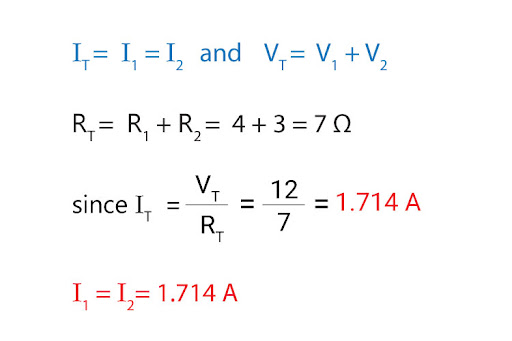Then, it is simply a matter of solving individually for potential difference: